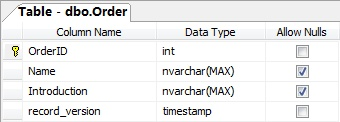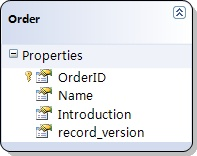Hello World
Spiga

## 在Linq to Sql中管理并发更新时的冲突（3）：使用记录的时间戳进行检测

2007-11-23 09:21 by 老赵, 5755 visits`try{    LinqToSqlDemoDataContext dataContext = new LinqToSqlDemoDataContext();    Order order = dataContext.Orders.Single(o => o.OrderID == 1);    order.Name = "New Order Name";    dataContext.Log = Console.Out;    // 在下面的语句上设置一个断点    dataContext.SubmitChanges();}catch (ChangeConflictException e){    Console.WriteLine(e.Message);}Console.ReadLine();`

`UPDATE [dbo].[Order]SET [Name] = @p2WHERE ([OrderID] = @p0) AND ([record_version] = @p1)SELECT [t1].[record_version]FROM [dbo].[Order] AS [t1]WHERE ((@@ROWCOUNT) > 0) AND ([t1].[OrderID] = @p3)-- @p0: Input Int (Size = 0; Prec = 0; Scale = 0) -- @p1: Input Timestamp (Size = 8; Prec = 0; Scale = 0) [SqlBinary(8)]-- @p2: Input NVarChar (Size = 14; Prec = 0; Scale = 0) [New Order Name]-- @p3: Input Int (Size = 0; Prec = 0; Scale = 0) -- Context: SqlProvider(Sql2005) Model: AttributedMetaModel Build: 3.5.21022.8Row not found or changed.`

`try{    LinqToSqlDemoDataContext dataContext = new LinqToSqlDemoDataContext();    Order order1 = dataContext.Orders.Single(o => o.OrderID == 1);    Order order2 = dataContext.Orders.Single(o => o.OrderID == 2);    Order order3 = dataContext.Orders.Single(o => o.OrderID == 3);    Console.WriteLine('Order 1: ' + order1.Introduction);    Console.WriteLine('Order 2: ' + order2.Introduction);    Console.WriteLine('Order 3: ' + order3.Introduction);    Console.WriteLine();    order1.Introduction = 'Order 1 modified.';    order2.Introduction = 'Order 2 modified.';    order3.Introduction = 'Order 3 modified.';    dataContext.Log = Console.Out;    // 在下面的语句上设置一个断点    dataContext.SubmitChanges();}catch (ChangeConflictException e){    Console.WriteLine('---------- ' + e.Message + ' ----------');}LinqToSqlDemoDataContext db = new LinqToSqlDemoDataContext();Order o1 = db.Orders.Single(o => o.OrderID == 1);Order o2 = db.Orders.Single(o => o.OrderID == 2);Order o3 = db.Orders.Single(o => o.OrderID == 3);Console.WriteLine('Order 1: ' + o1.Introduction);Console.WriteLine('Order 2: ' + o2.Introduction);Console.WriteLine('Order 3: ' + o3.Introduction);Console.ReadLine();`

 OrderID Name Introduction record_version 1 Order 1 This is order 1 2 Order 2 This is order 2 3 Order 3 This is order 3

`UPDATE Order SET OrderID = "New Order 2" WHERE OrderID = 2`

`Order 1: This is order 1Order 2: This is order 2Order 3: This is order 3UPDATE [dbo].[Order]SET [Introduction] = @p2WHERE ([OrderID] = @p0) AND ([record_version] = @p1)SELECT [t1].[record_version]FROM [dbo].[Order] AS [t1]WHERE ((@@ROWCOUNT) > 0) AND ([t1].[OrderID] = @p3)-- @p0: Input Int (Size = 0; Prec = 0; Scale = 0) -- @p1: Input Timestamp (Size = 8; Prec = 0; Scale = 0) [SqlBinary(8)]-- @p2: Input NVarChar (Size = 26; Prec = 0; Scale = 0) [Order 1 modified.]-- @p3: Input Int (Size = 0; Prec = 0; Scale = 0) -- Context: SqlProvider(Sql2005) Model: AttributedMetaModel Build: 3.5.21022.8UPDATE [dbo].[Order]SET [Introduction] = @p2WHERE ([OrderID] = @p0) AND ([record_version] = @p1)SELECT [t1].[record_version]FROM [dbo].[Order] AS [t1]WHERE ((@@ROWCOUNT) > 0) AND ([t1].[OrderID] = @p3)-- @p0: Input Int (Size = 0; Prec = 0; Scale = 0) -- @p1: Input Timestamp (Size = 8; Prec = 0; Scale = 0) [SqlBinary(8)]-- @p2: Input NVarChar (Size = 26; Prec = 0; Scale = 0) [Order 2 modified.]-- @p3: Input Int (Size = 0; Prec = 0; Scale = 0) -- Context: SqlProvider(Sql2005) Model: AttributedMetaModel Build: 3.5.21022.8---------- 1 of 2 updates failed. ----------Order 1: This is order 1Order 2: This is order 2Order 3: This is order 3`

# 相关文章### 19 条回复

1. 链接

好文！正需要：）：

2. 链接

#### henry2007-11-23 08:54:00

如何知道这个并发是不是存在冲突?
在现实中往往要人手去协调因为A和B需要更新一条记录,但数据的逻辑合法性到底是A正确还是B正确程序几乎是不可能知道.
对于所谓的更新冲突在多个appserver中的client是如何协调的?

3. 链接

#### 老赵2007-11-23 09:33:00

@henry
更新冲突并不代表更新的逻辑是不合法的，A和B都可以更新，但是如果A更新了，B就必须在A的基础上再更新，否则就会出现并发冲突——是这个意思。

4. 链接

mark

5. 链接

#### henry2007-11-23 09:53:00

@Jeffrey Zhao
在一台appservice里保证后者在前者的基础上作修改并不难,难点是多台appservice直接对数据操作上如何保证这么干.如果不能保证操作切入在一个点上基本很难入手(如果多个client server的事务有排它那基本就省事很多了).

6. 链接

#### 韩现龙2007-11-23 09:55:00

也?老赵,你的文章地址怎么是英文显示的嘞?

7. 链接

#### 木野狐(Neil Chen)2007-11-23 09:56:00

@韩现龙
发布文章的时候有个选项的。允许自定义友好的地址。

8. 链接

好文，关注。

9. 链接

好文，支持楼主。

10. 链接

#### Enzo2007-11-23 16:37:00

@木野狐(Neil Chen)
原来如此

11. 链接

#### 上海搬家公司[未注册用户]2007-11-23 18:42:00

如果多个client server的事务有排它那基本就省事很多了

12. 链接

#### Ariel Y.2008-03-02 21:58:00

"Sql Server中的timestamp类型和binary(8)在存储上非常类似（不过nullable的timestamp和nvarchar(8)类似）"

这个好像有点问题，nvarchar(8)应为varbinary(8)。

MSDN原文：A nonnullable timestamp column is semantically equivalent to a binary(8) column. A nullable timestamp column is semantically equivalent to a varbinary(8) column.

13. 链接

@Ariel Y.
对的，是我写错了。

14. 链接

#### 优雅旋律2008-05-23 10:55:00

请教下
到底是通过record_version的特定列名来标识其时间戳列还是通过类型timestamp
如果是通过类型的话 那假如有n个timestamp列
到底是使用的哪个?

15. 链接

#### 老赵2008-05-23 11:12:00

通过类型是timestamp，一个表为什么要有多个字段是timestamp？

16. 链接

#### 优雅旋律2008-05-25 00:07:00

忽然想到的
万一有n个字段为timestamp
会不会出bug.....

17. 链接

@优雅旋律
不能吧

18. 链接

#### 唐文2008-08-22 10:48:00

一个表只能有一个时间戳列。

19. 链接

下一篇呢？？？？？？？？